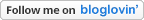## Question

Come back and visit soon!

## Wednesday, July 15, 2015

### Learn Math Fast System Review - How It Was Able to Teach My High School Student What Other Math Curricula Couldn't

In the fall of 2014, we switched my high school son’s math program. As we were finishing up the school year, he told me that he was struggling with math. My son who had previously received A type grades in math had not only dropped his grade by two levels, but his confidence in his math skills as well.

Since I had experience using Volume I of the Learn Math Fast System curriculum with my youngest (you can read my review about it here), and having been really impressed with it, I decided to try it with my oldest. Because my son hopes to get into the mechanical engineering field someday, I wanted a math program that would help him understand math, in this case Algebra.

This summer I had him begin the Learn Math Fast System Volume III, Pre-Algebra book as a refresher and to get him used to J.K. Mergens’ (the book's author) method of teaching. In addition, I wanted to make sure that we covered any gaps he may have had from using his previous math programs.

Volume III includes 25 lessons covering topics such as, “solving for x, ratios, proportions, exponents, square roots, terms, expressions, like terms, order of operations, distributive property of multiplication, graphs, linear equations, the y-intercept, and the slope formula.” All of the solutions to the problems are found at the end of the book.

My son had difficulty understanding how to do the slope formula problems with his old math program, but after using the Learn Math Fast System book he was able to do these types of problems without any difficulties. Also, since the lessons were short, he was able to finish the book within about a four week period (doing two lessons a day).

Next week my son will be completing Volume V of the Learn Math Fast System book which covers “Algebra I with a special focus on quadratic equations and formulas.” It includes 36 lessons, 7 chapter review tests, and a final test. Topics in this book include: “terminology, absolute value, terms with exponents, simplifying expressions, multiplying and dividing terms with exponents, square roots, cube roots, Laws of Algebra, multiplying polynomials, factoring polynomials, factoring out a common factor, prime factorization, quadratic equations, a perfect square, completing the square, quadratic formula, and intro to functions.”

Most of the lessons could be completed in less than an hour, which allowed him to go through two lessons a day. If the lesson was long, he would only do one lesson. Some of the review tests I would have him complete in one day, others I would allow him to take two days to complete.

According to the information on the curriculum's website, this book can be completed within a three to four month period. I would estimate that it has taken my son about three months to finish this book. (His last few lessons will be completed next week.)

I am happy to report that not only did his grade increase to an A, but his confidence in math is back again!

The Learn Math Fast System books have by far been my favorite math curriculum for the following reasons:

·         It provides step-by-step instructions on how to do the problems and includes a good amount of examples.
·         It is written in an easy-to-understand manner.
·         There aren’t hundreds of problems needing to be completed.
·         I can email (or call) J.K., the author, with any questions and receive a response within 24 hours.
·         The subject can be learned in half the time it would take with other math programs.
·       The high school student can complete this curriculum independently.

My son will be moving on to Volume VI, Algebra 2, as I prefer to have him continue with Algebra while it’s still fresh in his mind and then he will move on to Pre-Geometry (Volume IV) and Geometry (Volume VII).

If you are looking for a math curriculum for your student written in an easy-to-understand manner, then I highly recommend you try any of the Learn Math Fast System books.

By the way, if you’d like to try this curriculum use the code SLICE to get \$5.00 off your order (expires 12/31/15).

I know your time is precious, so any comment you share below will be greatly valued.

If you enjoyed this post, please Pin or Share it now by clicking on the icons below. Don't miss any of my posts. Become a subscriber by clicking on the Bloglovin' button or the RSS button below.